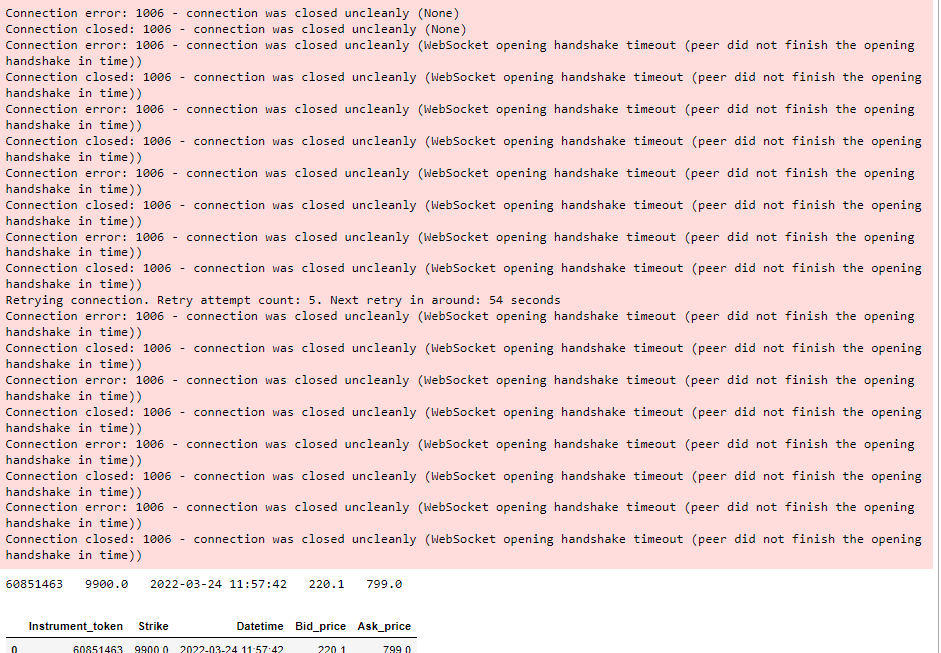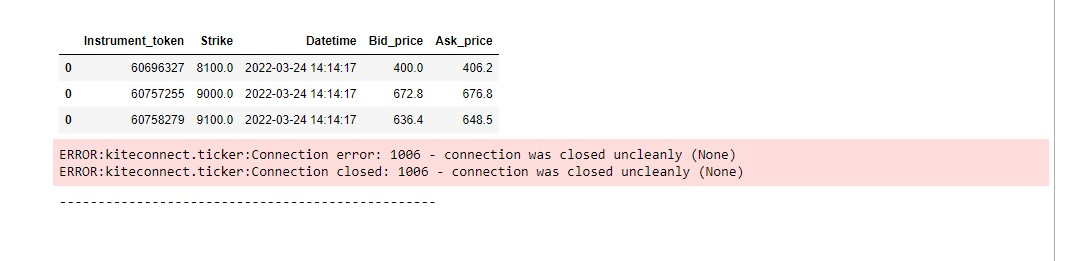# Connection error: 1006 - connection was closed uncleanly (WebSocket opening handshake timeout (peer

I'm getting this error, but my code still trying to reconnect, my internet connection is also good.
This is error which is coming time to time-but after these errors my code again start working, but during these errors my code didn't fetched at that time all instrument tokens values.
``from kiteconnect import KiteConnectimport timefrom kiteconnect import KiteTickerkws = KiteTicker(zerodha_api_key,acc_tkn)tokens = instrument_tokenprint(tokens)print(strike)# dict={9410818:'BANKNIFTY22MAR27000CE',9411074:'BANKNIFTY22MAR27000PE'}def conditions(ticks):    for i in range(len(ticks)):        instrumnet_token = ticks[i]['instrument_token']        if instrumnet_token in tokens:            index_number = tokens.index(instrumnet_token)            strike_price = strike[index_number]                         datetime = ticks[i]['exchange_timestamp']            bid_price = ticks[i]['depth']['buy']['price']            ask_price = ticks[i]['depth']['sell']['price']                        print(instrumnet_token, " ", strike_price, " ", datetime, " ", bid_price, " ", ask_price)                        output_df = pd.DataFrame(columns = ['Instrument_token', 'Strike', 'Datetime', 'Bid_price', 'Ask_price'])            c = 0            output_df.loc[c] = [instrumnet_token, strike_price, datetime, bid_price, ask_price]            display(output_df)        else:            pass        print("-------------------------------------------------")    print("\n\n")                def on_ticks(ws, ticks):    ticks = (ticks)    conditions(ticks)         def on_connect(ws, response):    ws.subscribe(tokens)    ws.set_mode(ws.MODE_FULL,tokens)kws.on_ticks = on_tickskws.on_connect = on_connectkws.connect(threaded=True)count=0while True:    count+=1    if(count%2==0):        if kws.is_connected():            kws.set_mode(kws.MODE_FULL,tokens)        else:            if kws.is_connected():                kws.set_mode(kws.MODE_FULL,tokens)        time.sleep(1)``
And This is my code.
What should I do?
Tagged:
• ``logging.basicConfig(level=logging.DEBUG)kws = KiteTicker(zerodha_api_key,acc_tkn)tokens = instrument_tokenprint(tokens)print(strike)def on_ticks(ws, ticks):    logging.debug("Ticks: {}".format(ticks))def on_connect(ws, response):    ws.subscribe(tokens)    ws.set_mode(ws.MODE_FULL, tokens)def on_close(ws, code, reason):     ws.stop()kws.on_ticks = on_tickskws.on_connect = on_connectkws.on_close = on_closekws.connect(threaded=True)while True:   #Perform required data operation using tick data    def on_ticks(ws, ticks):        helper_method(ticks)    def helper_method(ticks):        dataframes = []        for i in range(len(ticks)):            instrumnet_token = ticks[i]['instrument_token']            if instrumnet_token in tokens:                index_number = tokens.index(instrumnet_token)                strike_price = strike[index_number]                 datetime = ticks[i]['exchange_timestamp']                bid_price = ticks[i]['depth']['buy']['price']                ask_price = ticks[i]['depth']['sell']['price']                print(instrumnet_token, " ", strike_price, " ", datetime, " ", bid_price, " ", ask_price)                output_df = pd.DataFrame(columns = ['Instrument_token', 'Strike', 'Datetime', 'Bid_price', 'Ask_price'])                c = 0                output_df.loc[c] = [instrumnet_token, strike_price, datetime, bid_price, ask_price]                dataframes.append(output_df)            else:                pass            print("-------------------------------------------------")        df = pd.concat(dataframes)        display(df)        print("\n\n")    #Assign callback    kws.on_ticks=on_ticks  ``
@SRIJAN is it fine?
Still, I'm getting the same error. as well as now
• @SRIJAN Sir, but I'm getting an error in my code.